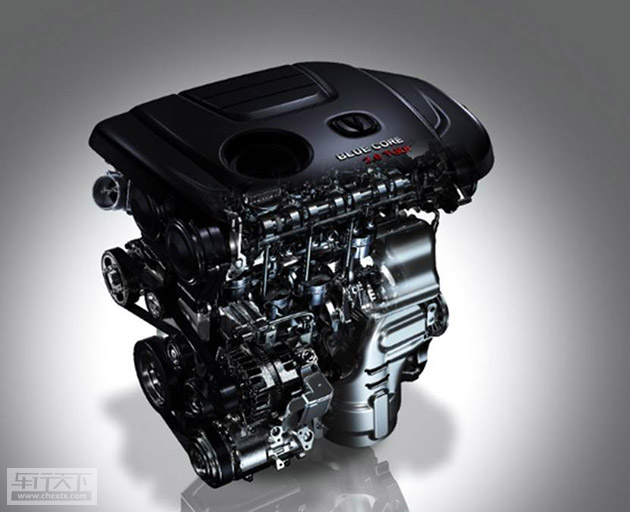马力和速度来证明品质 长安CS95完胜秘籍

2017年01月10日 16:04 来源：www.chextx.com 超过：83168次关注

梦想有一跃而起的惊喜，梦想拥有说走就走旅行，座驾的动力配备是实现梦想的前提条件。作为长安汽车品牌CS家族中最完美的SUV,长安CS95车型一经亮相，便成经典。

除了其来自意大利时尚设计之都——都灵设计中心别具一格的硬朗外型设计以外，英国伯明翰动力研发中心和美国底特律底盘研发中心的顶尖全球智慧，协同博世、博格华纳、爱信等全球顶级的供应商伙伴，历时6年，2000多个昼夜，倾力打造了这款“高性能全尺寸旗舰SUV”。强劲动力是高性能的外在体现，长安CS95的动力组成，是最为吸引人的亮点，是成就品质座驾的最强三剑客。

蓝鲸2.0TGDI发动机作为CS95车型的心脏，成功的运用了新技术——TC-废气涡轮增压技术，致使功率提升50%以上、中低速扭矩提升50%以上、整车油耗降低10-15%、是降低排放水平的关键；并显著提升驾驶推背感，发动机在1500rmp即可明显感觉到扭矩迅速提升，相比同级车型扭矩提升更快。

而在提高整车舒适性及发动机的可靠性方面，蓝鲸D20TGDI发动机应用的平衡轴技术，可最大限度的降低发动机运行不平衡的因素，改善一般的直列四缸发动机，在运行时，二阶往复惯性力不平衡导致发动机振动大为驾乘人员带来的不舒适性。

长安CS95车型的最大功率171Kw，在1750rpm-3500rpm之间持续爆发360N·m的最大扭矩，已经达到了与国际品牌大型SUV发动机的同级水平。发动机一经启动，犹如一条蓝鲸一摆尾鳍，驱动身体充满力量的向前行进，彰显了瞬间爆发力和强大的力量感。如此强大的发动机设备，瞬间秒杀了大部分主流合资车型。长安CS95所搭载的爱信TF-81SC 6AT变速箱，最大可承载450N·m的扭矩，具有传递效率高效、燃油经济性、控制功能丰富等特点，快速换挡可以在0.8秒之内实现响应。就如同蓝鲸2.0TGDI发动机强大的消化系统，能够迅速消化动力能量，提升加速度。让蓝鲸犹如在浩瀚大海中，吃饱之后全力而行，迅猛而灵敏。长安CS95的四驱车型所采用的国际领先的博格华纳全新一代NexTrac 智能四驱电子控制系统，具备强大的越野持续脱困能力。无论是蜿蜒盘行的山间，还是沟坎从生的险路，都能够如履平地。它可以根据道路状况合理的分配前后轮扭矩，同时为车辆带来更高效的驱动性能和起步加速性能。如蓝鲸的左膀右臂般，配合整个动力系统，支持庞大的身躯畅行无阻。“蓝鲸2.0TGDI发动机+爱信TF-81SC 6AT变速箱+博格华纳NexTrac四驱系统”，就是长安CS95车型的动力三剑客，是整车高性能的核心力量，是提供前行的重要动力。试想一下，当您驾驶着长安CS95车型，强大的动力系统不仅为您节省了行驶时间，还为您节约了不少的自驾成本。拥有这样的一款座驾，能够让您体验到高性能动力系统所带来的驾乘舒适感和对速度的追求。

作为中国汽车品牌的扛鼎之作，长安CS95以强劲的动力和速度展现了高品质汽车的高性能特点，用自主研发的一流尖端技术完胜了主流合资品牌车型，填补国内2.0L及以上增压直喷发动机自主开发能力空白，用国内最强，世界领先的发动机系统，证明了中国力量。

相关文章

0-500 字已有评论 0条 查看评论>>

﻿
• 快速找车
• 选择品牌
• 选择品牌
• A  奥迪
• A  阿斯顿·马丁
• A  阿尔法·罗密欧
• B  宝沃
• B  布加迪
• B  巴博斯
• B  保时捷
• B  宾利
• B  奔驰
• B  宝马
• B  本田
• B  别克
• B  标致
• B  比亚迪
• B  宝骏
• B  北汽制造
• B  北汽新能源
• B  北汽幻速
• B  北汽威旺
• B  北京汽车
• B  奔腾
• B  北汽绅宝
• C  长安
• C  长安商用
• C  长城
• C  昌河
• D  大众
• D  道奇
• D  DS
• D  东南
• D  东风风神
• D  东风风行
• D  东风小康
• D  东风风度
• D  东风
• F  福特
• F  丰田
• F  菲亚特
• F  法拉利
• F  福田
• F  福迪
• F  福汽启腾
• G  观致
• G  广汽传祺
• G  广汽吉奥
• G  GMC
• H  红旗
• H  汉腾汽车
• H  哈弗
• H  哈飞
• H  海格
• H  海马
• H  华颂
• H  黄海
• H  华泰
• H  恒天
• J  吉利汽车
• J  捷豹
• J  Jeep
• J  江淮
• J  江铃
• J  金杯
• J  九龙
• J  金旅
• K  凯翼
• K  凯迪拉克
• K  克莱斯勒
• K  科尼塞克
• K  卡威
• K  开瑞
• L  路虎
• L  林肯
• L  劳斯莱斯
• L  兰博基尼
• L  雷克萨斯
• L  铃木
• L  雷诺
• L  理念
• L  力帆
• L  莲花汽车
• L  猎豹
• L  路特斯
• L  陆风
• M  马自达
• M  MG
• M  MINI
• M  玛莎拉蒂
• M  摩根
• M  迈凯轮
• N  纳智捷
• O  欧宝
• O  讴歌
• O  欧朗
• Q  奇瑞
• Q  起亚
• Q  启辰
• R  日产
• R  荣威
• R  瑞麒
• S  三菱
• S  斯威汽车
• S  萨博
• S  smart
• S  斯柯达
• S  斯巴鲁
• S  思铭
• S  双龙
• S  上汽大通
• S  双环
• T  特斯拉
• T  腾势
• W  沃尔沃
• W  五菱汽车
• W  五十铃
• W  威兹曼
• W  威麟
• X  现代
• X  雪佛兰
• X  雪铁龙
• X  西雅特
• Y  一汽
• Y  英菲尼迪
• Y  英致
• Y  依维柯
• Y  野马汽车
• Y  永源
• Z  众泰
• Z  中华
• Z  中兴
• Z  知豆
• 选择车系
• 选择车系
• 车型对比
• 选择品牌
• 选择品牌
• A  奥迪
• A  阿斯顿·马丁
• A  阿尔法·罗密欧
• B  宝沃
• B  布加迪
• B  巴博斯
• B  保时捷
• B  宾利
• B  奔驰
• B  宝马
• B  本田
• B  别克
• B  标致
• B  比亚迪
• B  宝骏
• B  北汽制造
• B  北汽新能源
• B  北汽幻速
• B  北汽威旺
• B  北京汽车
• B  奔腾
• B  北汽绅宝
• C  长安
• C  长安商用
• C  长城
• C  昌河
• D  大众
• D  道奇
• D  DS
• D  东南
• D  东风风神
• D  东风风行
• D  东风小康
• D  东风风度
• D  东风
• F  福特
• F  丰田
• F  菲亚特
• F  法拉利
• F  福田
• F  福迪
• F  福汽启腾
• G  观致
• G  广汽传祺
• G  广汽吉奥
• G  GMC
• H  红旗
• H  汉腾汽车
• H  哈弗
• H  哈飞
• H  海格
• H  海马
• H  华颂
• H  黄海
• H  华泰
• H  恒天
• J  吉利汽车
• J  捷豹
• J  Jeep
• J  江淮
• J  江铃
• J  金杯
• J  九龙
• J  金旅
• K  凯翼
• K  凯迪拉克
• K  克莱斯勒
• K  科尼塞克
• K  卡威
• K  开瑞
• L  路虎
• L  林肯
• L  劳斯莱斯
• L  兰博基尼
• L  雷克萨斯
• L  铃木
• L  雷诺
• L  理念
• L  力帆
• L  莲花汽车
• L  猎豹
• L  路特斯
• L  陆风
• M  马自达
• M  MG
• M  MINI
• M  玛莎拉蒂
• M  摩根
• M  迈凯轮
• N  纳智捷
• O  欧宝
• O  讴歌
• O  欧朗
• Q  奇瑞
• Q  起亚
• Q  启辰
• R  日产
• R  荣威
• R  瑞麒
• S  三菱
• S  斯威汽车
• S  萨博
• S  smart
• S  斯柯达
• S  斯巴鲁
• S  思铭
• S  双龙
• S  上汽大通
• S  双环
• T  特斯拉
• T  腾势
• W  沃尔沃
• W  五菱汽车
• W  五十铃
• W  威兹曼
• W  威麟
• X  现代
• X  雪佛兰
• X  雪铁龙
• X  西雅特
• Y  一汽
• Y  英菲尼迪
• Y  英致
• Y  依维柯
• Y  野马汽车
• Y  永源
• Z  众泰
• Z  中华
• Z  中兴
• Z  知豆
• 选择车系
• 选择车系
• 选择车型
• 选择车型
• 意见反馈